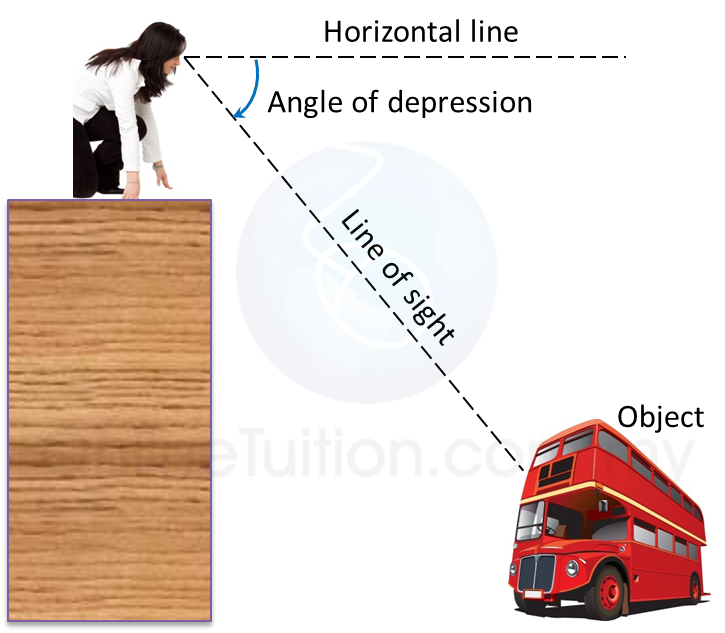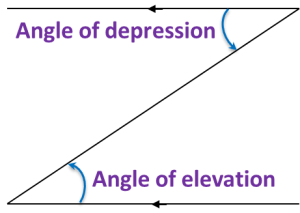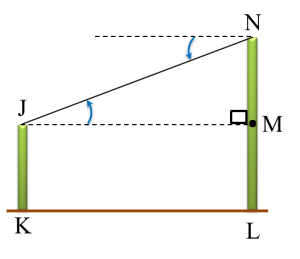# 10.1 Angles of Elevation and Depression

10.1 Angle of Elevation and Angle of Depression

1. Angle of elevation is the acute angle measured from a horizontal line up to the line of sight.Example 1:Angle of elevation of object O from P = ∠OPA

2. Angle of depression is the acute angle measured down from a horizontal line to the line of sight.Example 2:Angle of depression of object O from P = ∠OPA

3. The angle of elevation or angle of depression is always measured from the horizontal line.Example 3:
Diagram below shows two vertical poles JK and NL on a horizontal plane. is a point on NL such that JK = ML.The angle of depression of point J from point is

Solution:
The angle of depression of point J from N is the angle between line JN and the horizontal line through N.Angle of depression of J from N
= angle of elevation of N from J
=∠NJM